jbera
Multivariate residual normality test.
Syntax
var_name.jbera(options)
You must specify a factorization method using the “factor=” option.
Options

 factor=chol Factorization by the inverse of the Cholesky factor of the residual covariance matrix. factor=cor Factorization by the inverse square root of the residual correlation matrix (Doornik and Hansen, 1994). factor=cov Factorization by the inverse square root of the residual covariance matrix (Urzua, 1997). name=arg Save the test statistics in a named matrix object. See below for a description of the statistics contained in the stored matrix. prompt Force the dialog to appear from within a program. p Print the test results.
The “name=” option stores the following matrix. Let the VAR have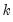endogenous variables. Then the stored matrix will have dimension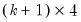. The firstrows contain statistics for each orthogonal component, where the first column contains the third moments, the second column contains the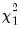statistics for the third moments, the third column contains the fourth moments, and the fourth column holds thestatistics for the fourth moments. The sum of the second and fourth columns are the Jarque-Bera statistics reported in the last output table.
The last row contains statistics for the joint test. The second and fourth column of the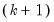row is simply the sum of all the rows above in the corresponding column and are the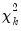statistics for the joint skewness and kurtosis tests, respectively. These joint skewness and kurtosis statistics add up to the joint Jarque-Bera statistic reported in the output table, except for the “factor=cov” option. When this option is set, the joint Jarque-Bera statistic includes all cross moments (in addition to the pure third and fourth moments). The overall Jarque-Bera statistic for this statistic is stored in the first column of therow (which will be a missing value for all other options).
Examples
sys01.jbera(factor=cor,name=jb)
carries out the residual multivariate normality test using the inverse square root of the residual correlation matrix as the factorization matrix and stores the results in a matrix named JB.
Cross-references
See “Vector Autoregression (VAR) Models” for a discussion of the test in the context of VAR diagnostics.